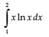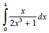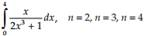### Create an Account

Home / Questions / Consider a Find the actual value of the integral analytically b Evaluate by executing ...

# Consider a Find the actual value of the integral analytically b Evaluate by executing the user-defined function Simpson Section 6.3 using n 4 c Evaluate by executing the user-defined fun

Considera. Find the actual value of the integral analytically.

b. Evaluate by executing the user-defined function Simpson (Section 6.3) using n = 4.

c. Evaluate by executing the user-defined function Gauss_ Quad_4 (see Problem 69).

d. Calculate the relative error in (b) and (c) and comment.

Problem 69

Write a user-defined function with syntax I = Gauss_ Quad_4(f,a,b) that evaluates the integral of f from a to b using the Gaussian quadrature with n = 4. Execute Gauss_Quad_4 to evaluate the integral in Problem 67:Problem 67

Using the Gaussian quadrature with the indicated number(s) of nodesJul 22 2020 View more View LessSubscribe To Get Solution